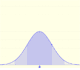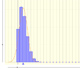# Calculating probabilities in Normal distributions

It may be interesting to familiarize ourselves with the probabilities correspondig to different intervals in normal distributions.

Two points on the x-axis determine the extremes of the interval for which the probability is calculated (approximately)

Different options A1, A2, ..., A6 correspond to different intervals that can be defined with those two points. Taken in pairs these are complementary in the sense that the sum of probabilities is 1.

We can modify the parameters of the normal distribution and see how the probabilities vary.

The gray dots control vertical and horizontal scales of the graphic. By pressing the right button and dragging we can move left and right.

REFERENCES

George Marsaglia's article Evaluating the Normal Distribution.